Скачать презентацию Production Operations Management Fundamentals of Inventory Management From

bc0b8f287850e954d9d5437d76f42923.ppt

• Количество слайдов: 51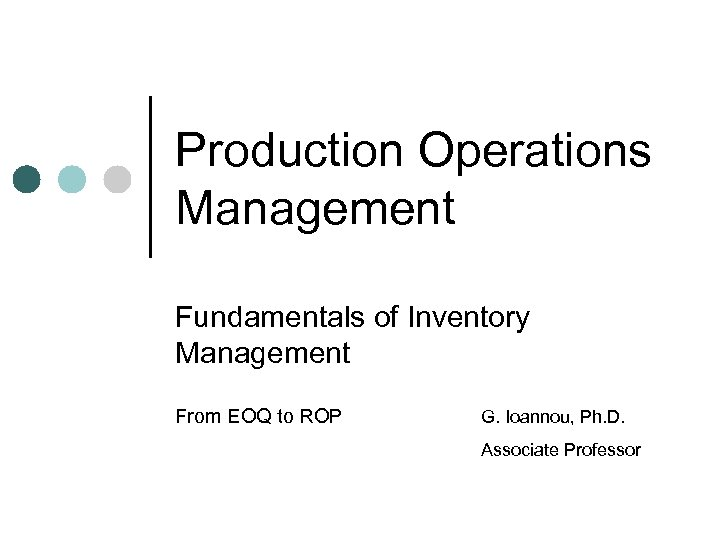Production Operations Management Fundamentals of Inventory Management From EOQ to ROP G. Ioannou, Ph. D. Associate Professor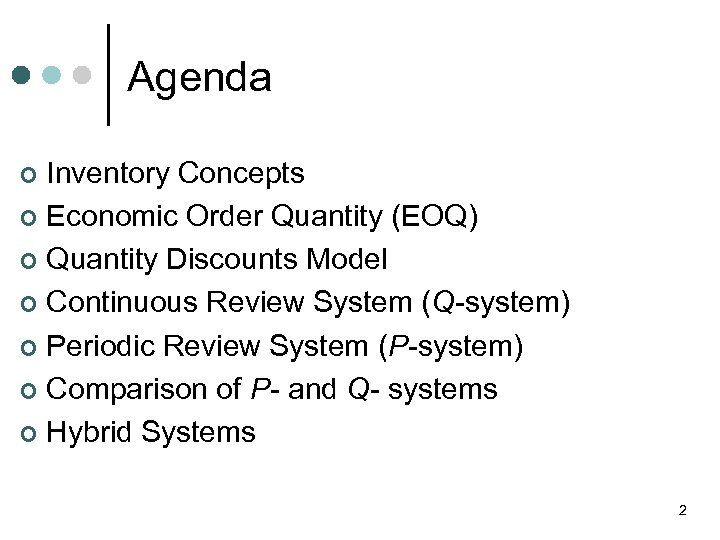Agenda Inventory Concepts ¢ Economic Order Quantity (EOQ) ¢ Quantity Discounts Model ¢ Continuous Review System (Q-system) ¢ Periodic Review System (P-system) ¢ Comparison of P- and Q- systems ¢ Hybrid Systems ¢ 2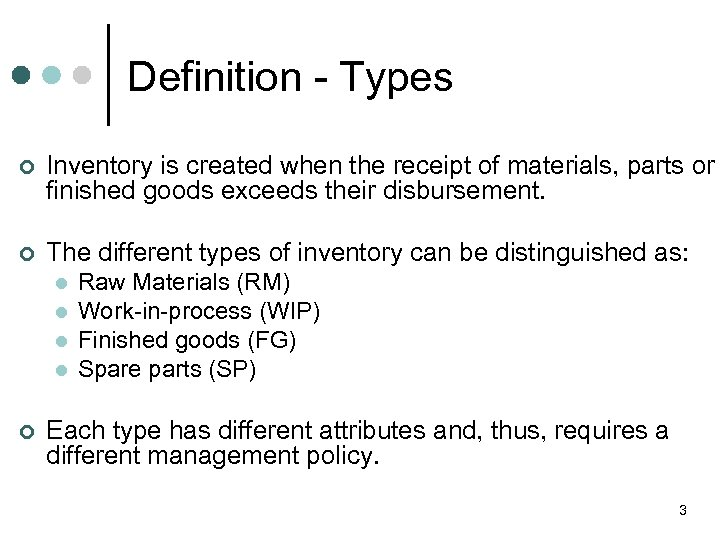Definition - Types ¢ Inventory is created when the receipt of materials, parts or finished goods exceeds their disbursement. ¢ The different types of inventory can be distinguished as: l l ¢ Raw Materials (RM) Work-in-process (WIP) Finished goods (FG) Spare parts (SP) Each type has different attributes and, thus, requires a different management policy. 3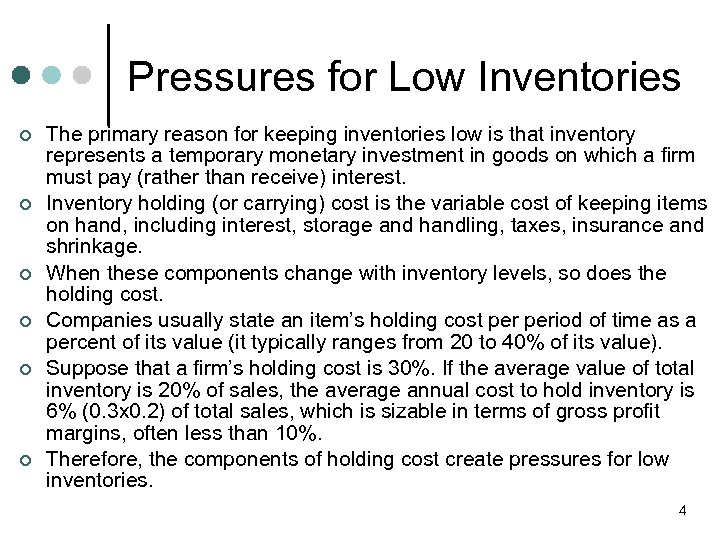Pressures for Low Inventories ¢ ¢ ¢ The primary reason for keeping inventories low is that inventory represents a temporary monetary investment in goods on which a firm must pay (rather than receive) interest. Inventory holding (or carrying) cost is the variable cost of keeping items on hand, including interest, storage and handling, taxes, insurance and shrinkage. When these components change with inventory levels, so does the holding cost. Companies usually state an item’s holding cost period of time as a percent of its value (it typically ranges from 20 to 40% of its value). Suppose that a firm’s holding cost is 30%. If the average value of total inventory is 20% of sales, the average annual cost to hold inventory is 6% (0. 3 x 0. 2) of total sales, which is sizable in terms of gross profit margins, often less than 10%. Therefore, the components of holding cost create pressures for low inventories. 4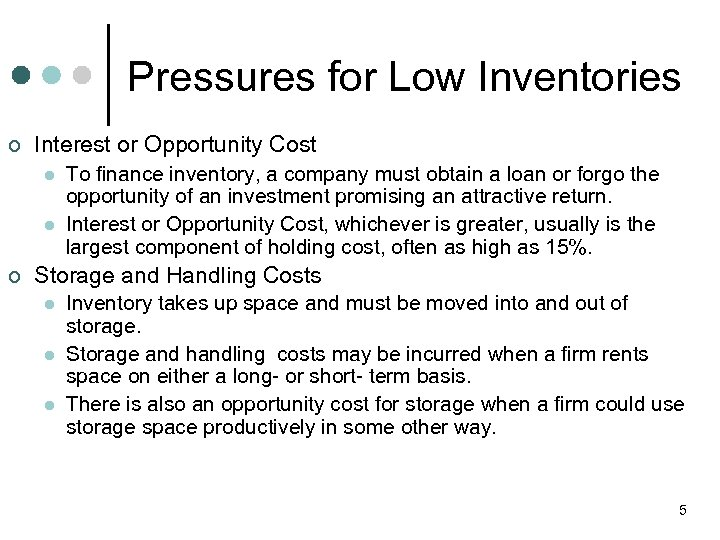Pressures for Low Inventories ¢ Interest or Opportunity Cost l l ¢ To finance inventory, a company must obtain a loan or forgo the opportunity of an investment promising an attractive return. Interest or Opportunity Cost, whichever is greater, usually is the largest component of holding cost, often as high as 15%. Storage and Handling Costs l l l Inventory takes up space and must be moved into and out of storage. Storage and handling costs may be incurred when a firm rents space on either a long- or short- term basis. There is also an opportunity cost for storage when a firm could use storage space productively in some other way. 5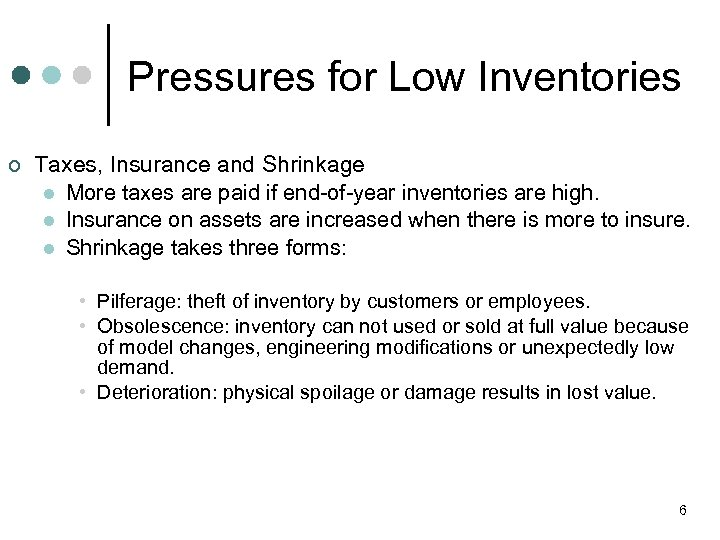Pressures for Low Inventories ¢ Taxes, Insurance and Shrinkage l More taxes are paid if end-of-year inventories are high. l Insurance on assets are increased when there is more to insure. l Shrinkage takes three forms: • Pilferage: theft of inventory by customers or employees. • Obsolescence: inventory can not used or sold at full value because of model changes, engineering modifications or unexpectedly low demand. • Deterioration: physical spoilage or damage results in lost value. 6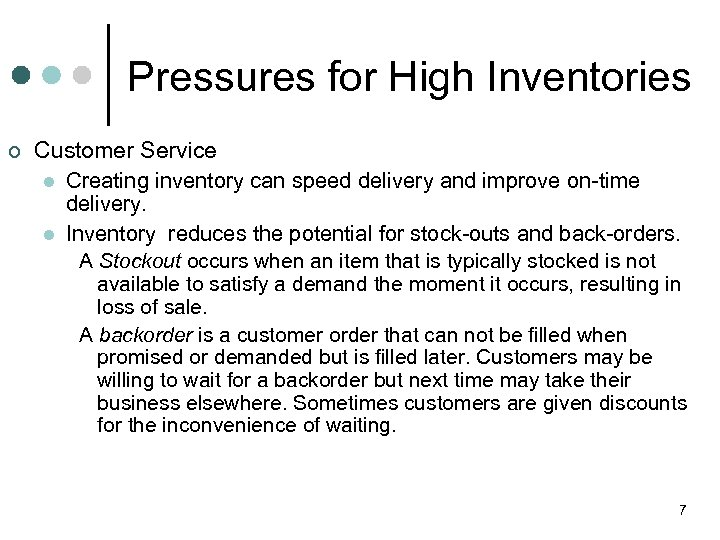Pressures for High Inventories ¢ Customer Service l Creating inventory can speed delivery and improve on-time delivery. l Inventory reduces the potential for stock-outs and back-orders. A Stockout occurs when an item that is typically stocked is not available to satisfy a demand the moment it occurs, resulting in loss of sale. A backorder is a customer order that can not be filled when promised or demanded but is filled later. Customers may be willing to wait for a backorder but next time may take their business elsewhere. Sometimes customers are given discounts for the inconvenience of waiting. 7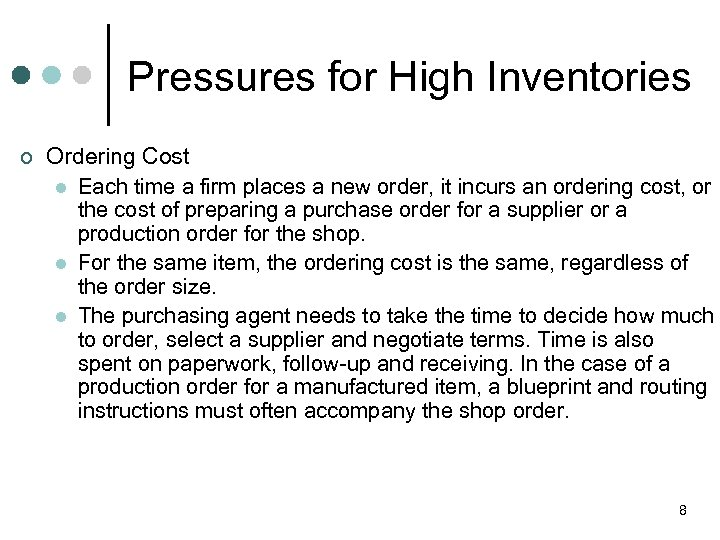Pressures for High Inventories ¢ Ordering Cost l Each time a firm places a new order, it incurs an ordering cost, or the cost of preparing a purchase order for a supplier or a production order for the shop. l For the same item, the ordering cost is the same, regardless of the order size. l The purchasing agent needs to take the time to decide how much to order, select a supplier and negotiate terms. Time is also spent on paperwork, follow-up and receiving. In the case of a production order for a manufactured item, a blueprint and routing instructions must often accompany the shop order. 8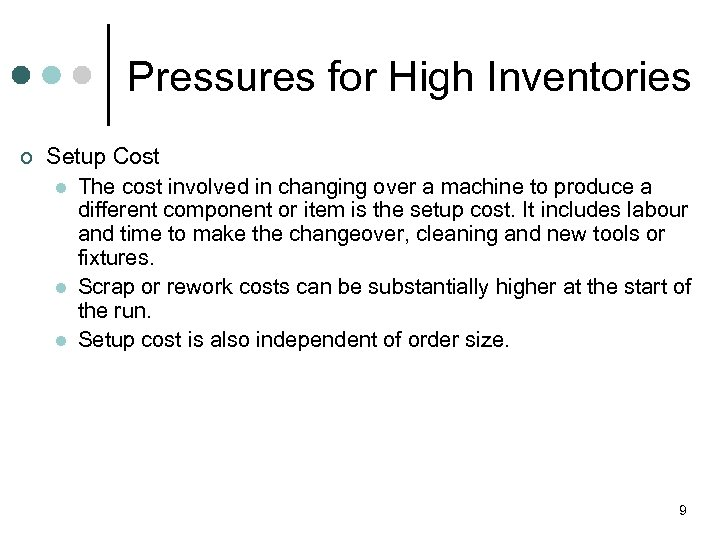Pressures for High Inventories ¢ Setup Cost l The cost involved in changing over a machine to produce a different component or item is the setup cost. It includes labour and time to make the changeover, cleaning and new tools or fixtures. l Scrap or rework costs can be substantially higher at the start of the run. l Setup cost is also independent of order size. 9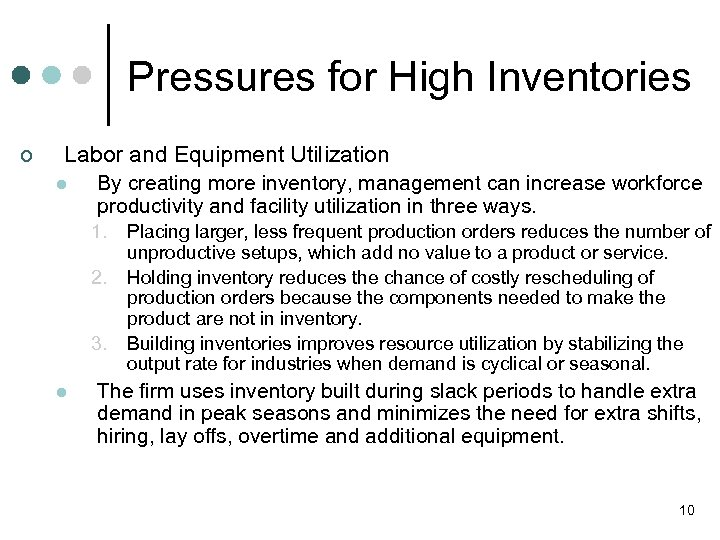Pressures for High Inventories ¢ Labor and Equipment Utilization l By creating more inventory, management can increase workforce productivity and facility utilization in three ways. 1. 2. 3. l Placing larger, less frequent production orders reduces the number of unproductive setups, which add no value to a product or service. Holding inventory reduces the chance of costly rescheduling of production orders because the components needed to make the product are not in inventory. Building inventories improves resource utilization by stabilizing the output rate for industries when demand is cyclical or seasonal. The firm uses inventory built during slack periods to handle extra demand in peak seasons and minimizes the need for extra shifts, hiring, lay offs, overtime and additional equipment. 10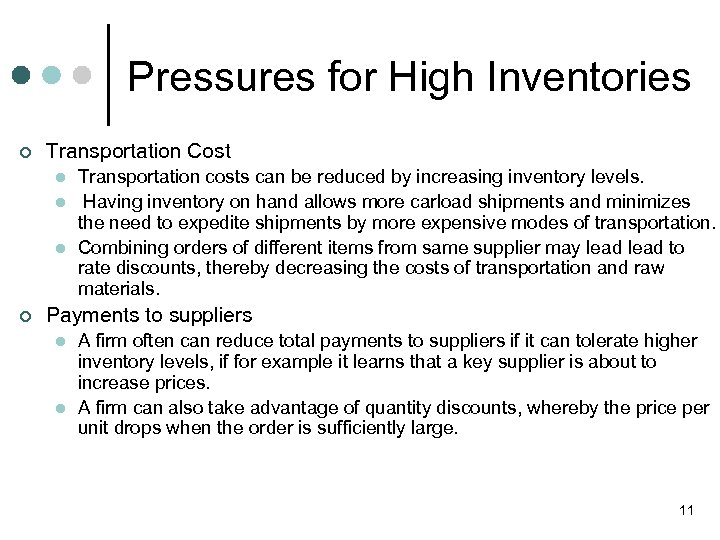Pressures for High Inventories ¢ Transportation Cost l l l ¢ Transportation costs can be reduced by increasing inventory levels. Having inventory on hand allows more carload shipments and minimizes the need to expedite shipments by more expensive modes of transportation. Combining orders of different items from same supplier may lead to rate discounts, thereby decreasing the costs of transportation and raw materials. Payments to suppliers l l A firm often can reduce total payments to suppliers if it can tolerate higher inventory levels, if for example it learns that a key supplier is about to increase prices. A firm can also take advantage of quantity discounts, whereby the price per unit drops when the order is sufficiently large. 11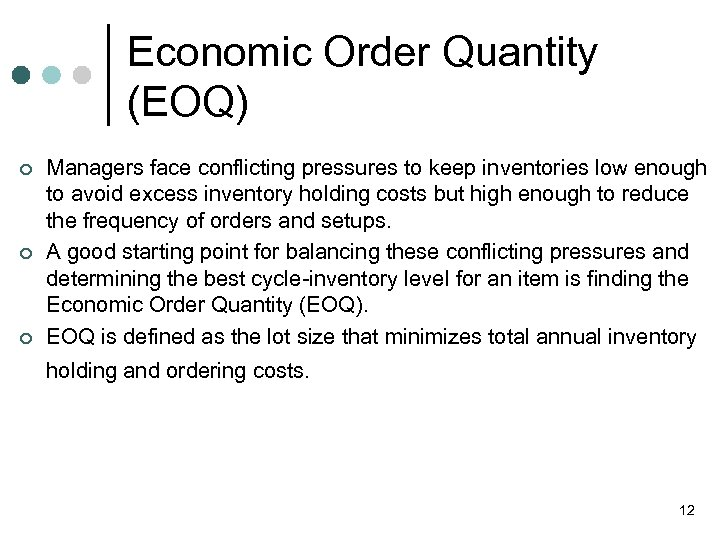Economic Order Quantity (EOQ) ¢ ¢ ¢ Managers face conflicting pressures to keep inventories low enough to avoid excess inventory holding costs but high enough to reduce the frequency of orders and setups. A good starting point for balancing these conflicting pressures and determining the best cycle-inventory level for an item is finding the Economic Order Quantity (EOQ). EOQ is defined as the lot size that minimizes total annual inventory holding and ordering costs. 12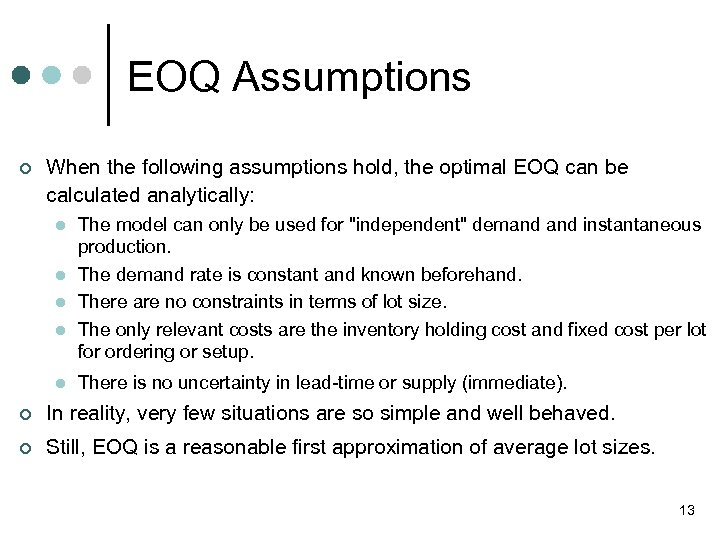EOQ Assumptions ¢ When the following assumptions hold, the optimal EOQ can be calculated analytically: l l l The model can only be used for "independent" demand instantaneous production. The demand rate is constant and known beforehand. There are no constraints in terms of lot size. The only relevant costs are the inventory holding cost and fixed cost per lot for ordering or setup. There is no uncertainty in lead-time or supply (immediate). ¢ In reality, very few situations are so simple and well behaved. ¢ Still, EOQ is a reasonable first approximation of average lot sizes. 13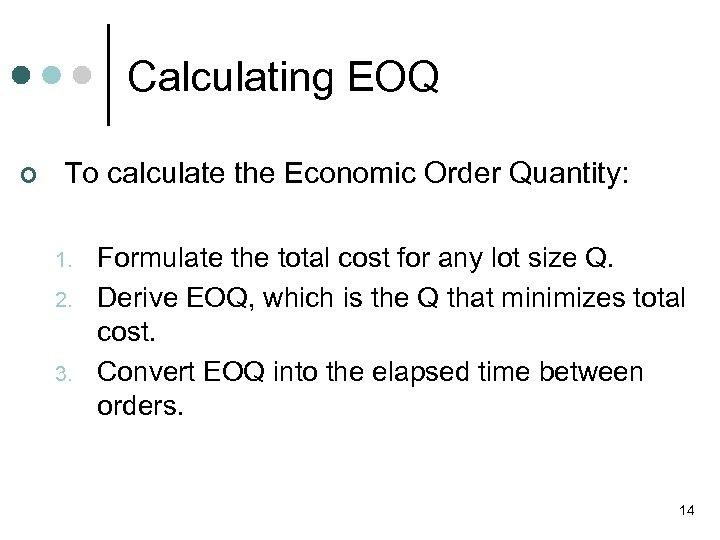Calculating EOQ ¢ To calculate the Economic Order Quantity: 1. 2. 3. Formulate the total cost for any lot size Q. Derive EOQ, which is the Q that minimizes total cost. Convert EOQ into the elapsed time between orders. 14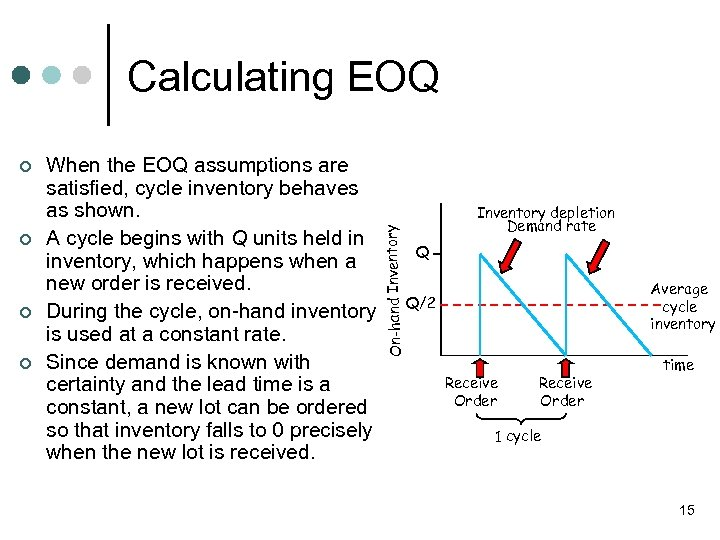¢ ¢ When the EOQ assumptions are satisfied, cycle inventory behaves as shown. A cycle begins with Q units held in inventory, which happens when a new order is received. During the cycle, on-hand inventory is used at a constant rate. Since demand is known with certainty and the lead time is a constant, a new lot can be ordered so that inventory falls to 0 precisely when the new lot is received. On-hand Inventory Calculating EOQ Inventory depletion Demand rate Q Average cycle inventory Q/2 Receive Order time 1 cycle 15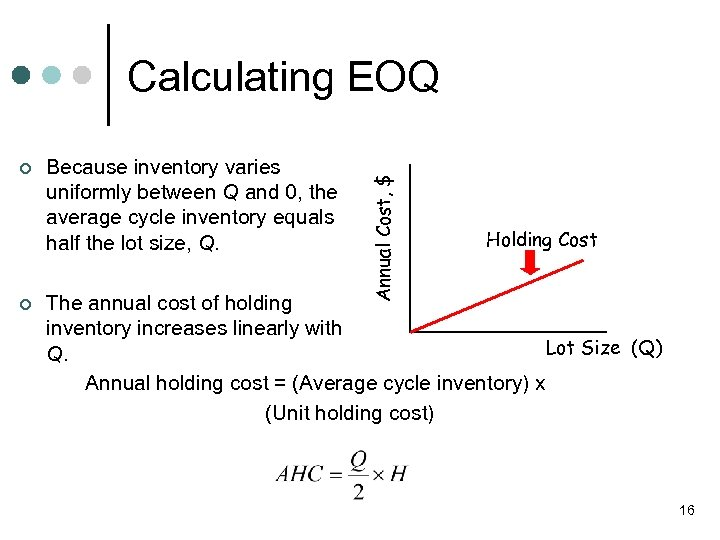¢ ¢ Because inventory varies uniformly between Q and 0, the average cycle inventory equals half the lot size, Q. Annual Cost, \$ Calculating EOQ Holding Cost The annual cost of holding inventory increases linearly with Lot Size (Q) Q. Annual holding cost = (Average cycle inventory) x (Unit holding cost) 16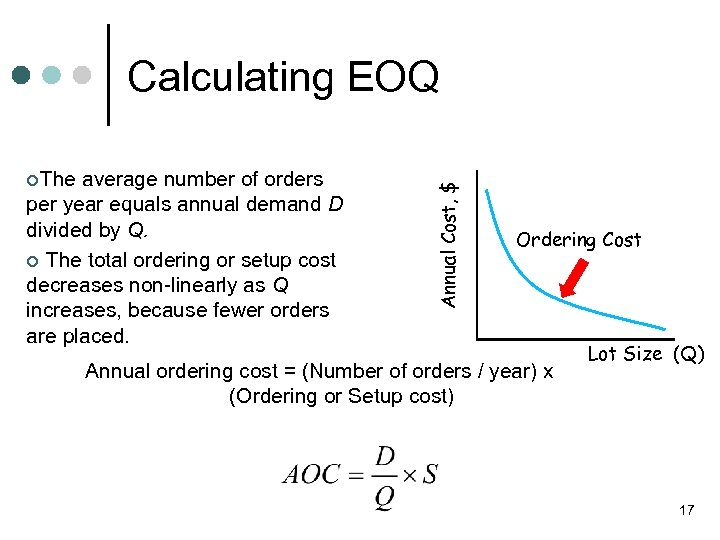¢The average number of orders per year equals annual demand D divided by Q. ¢ The total ordering or setup cost decreases non-linearly as Q increases, because fewer orders are placed. Annual Cost, \$ Calculating EOQ Ordering Cost Annual ordering cost = (Number of orders / year) x (Ordering or Setup cost) Lot Size (Q) 17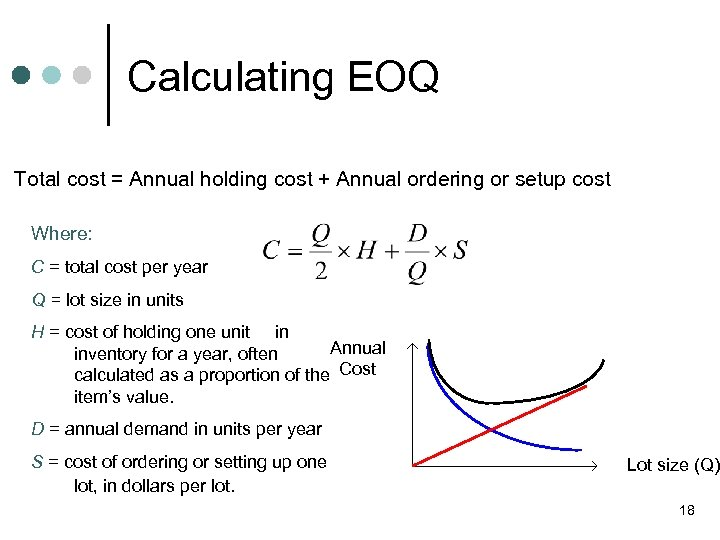Calculating EOQ Total cost = Annual holding cost + Annual ordering or setup cost Where: C = total cost per year Q = lot size in units H = cost of holding one unit in Annual inventory for a year, often calculated as a proportion of the Cost item’s value. D = annual demand in units per year S = cost of ordering or setting up one lot, in dollars per lot. Lot size (Q) 18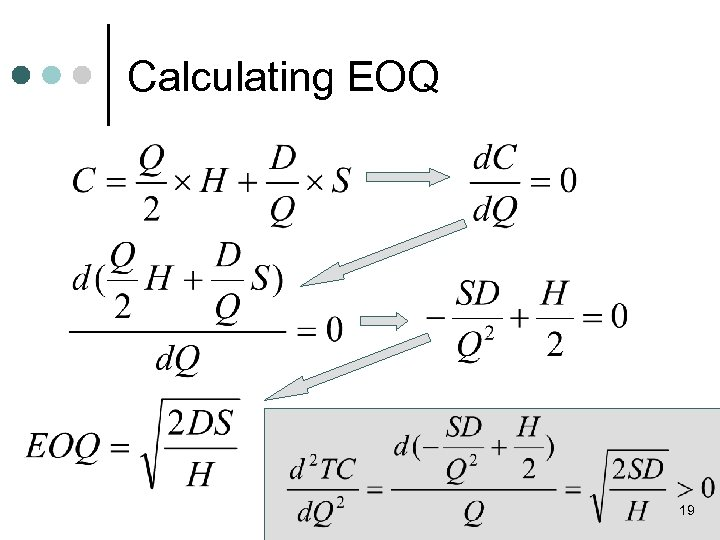Calculating EOQ 19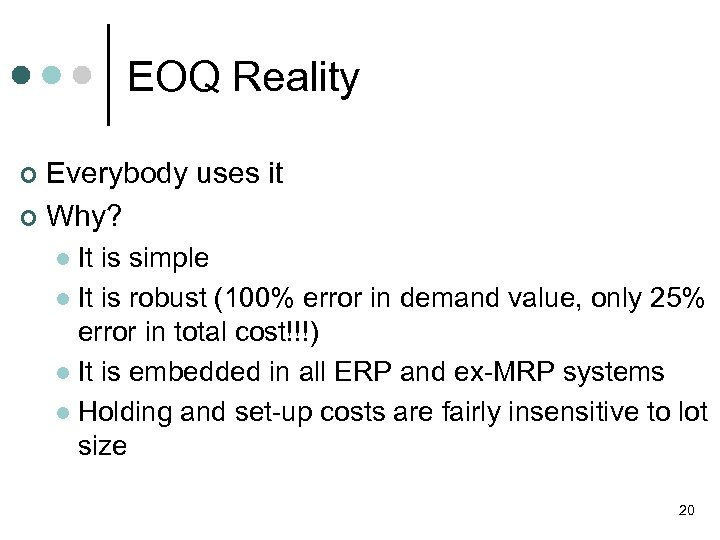EOQ Reality Everybody uses it ¢ Why? ¢ It is simple l It is robust (100% error in demand value, only 25% error in total cost!!!) l It is embedded in all ERP and ex-MRP systems l Holding and set-up costs are fairly insensitive to lot size l 20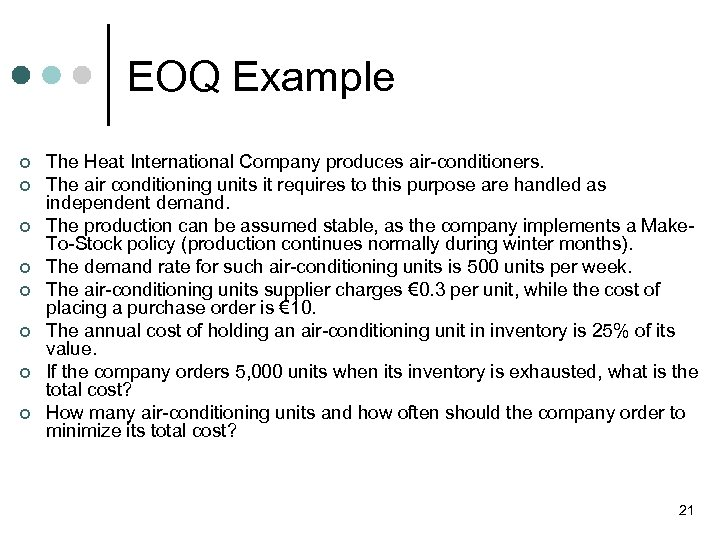EOQ Example ¢ ¢ ¢ ¢ The Heat International Company produces air-conditioners. The air conditioning units it requires to this purpose are handled as independent demand. The production can be assumed stable, as the company implements a Make. To-Stock policy (production continues normally during winter months). The demand rate for such air-conditioning units is 500 units per week. The air-conditioning units supplier charges € 0. 3 per unit, while the cost of placing a purchase order is € 10. The annual cost of holding an air-conditioning unit in inventory is 25% of its value. If the company orders 5, 000 units when its inventory is exhausted, what is the total cost? How many air-conditioning units and how often should the company order to minimize its total cost? 21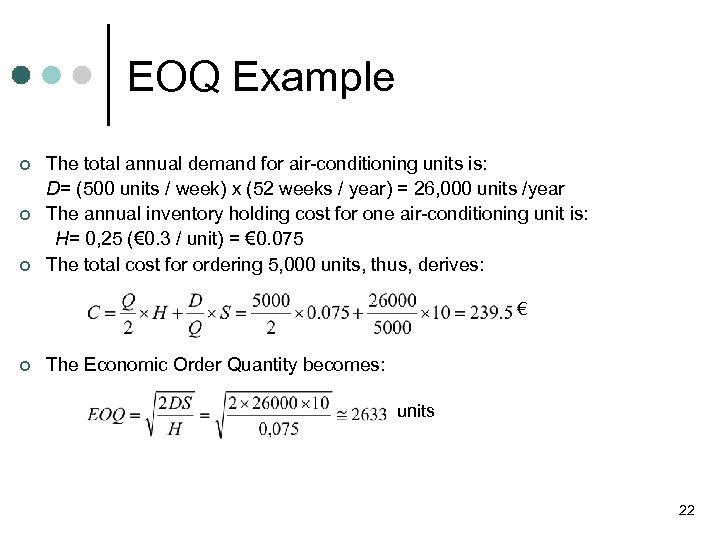EOQ Example ¢ ¢ ¢ The total annual demand for air-conditioning units is: D= (500 units / week) x (52 weeks / year) = 26, 000 units /year The annual inventory holding cost for one air-conditioning unit is: Η= 0, 25 (€ 0. 3 / unit) = € 0. 075 The total cost for ordering 5, 000 units, thus, derives: € ¢ The Economic Order Quantity becomes: units 22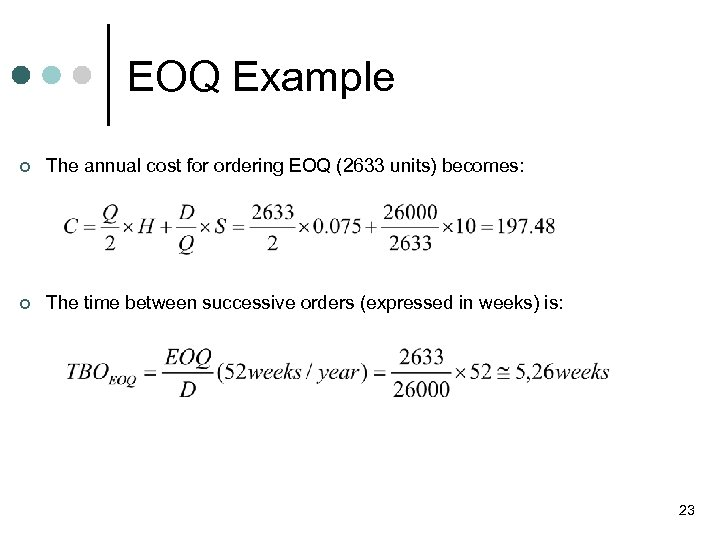EOQ Example ¢ The annual cost for ordering EOQ (2633 units) becomes: ¢ The time between successive orders (expressed in weeks) is: 23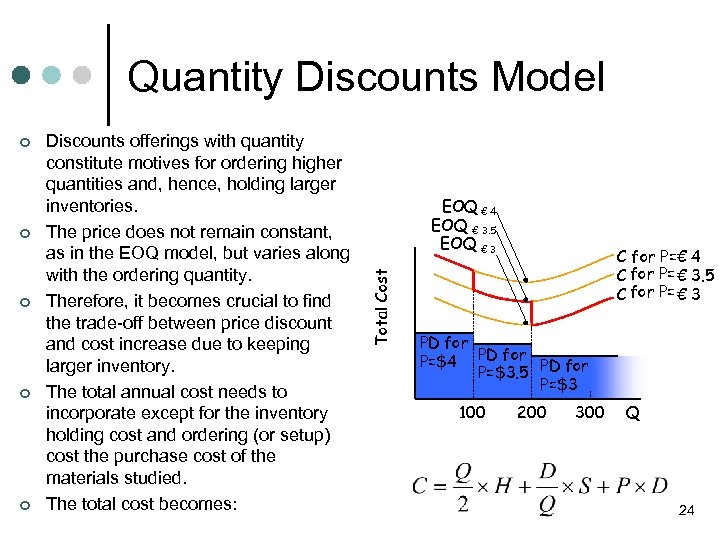Quantity Discounts Model ¢ ¢ Discounts offerings with quantity constitute motives for ordering higher quantities and, hence, holding larger inventories. The price does not remain constant, as in the EOQ model, but varies along with the ordering quantity. Therefore, it becomes crucial to find the trade-off between price discount and cost increase due to keeping larger inventory. The total annual cost needs to incorporate except for the inventory holding cost and ordering (or setup) cost the purchase cost of the materials studied. The total cost becomes: EOQ € 4 EOQ € 3. 5 EOQ € 3 Total Cost ¢ C for Ρ=€ 4 € C for Ρ= € 3. 5 C for Ρ= € 3 PD για for Ρ=\$4 PD για for Ρ=\$3. 5 Ρ=\$3 100 200 300 Q 24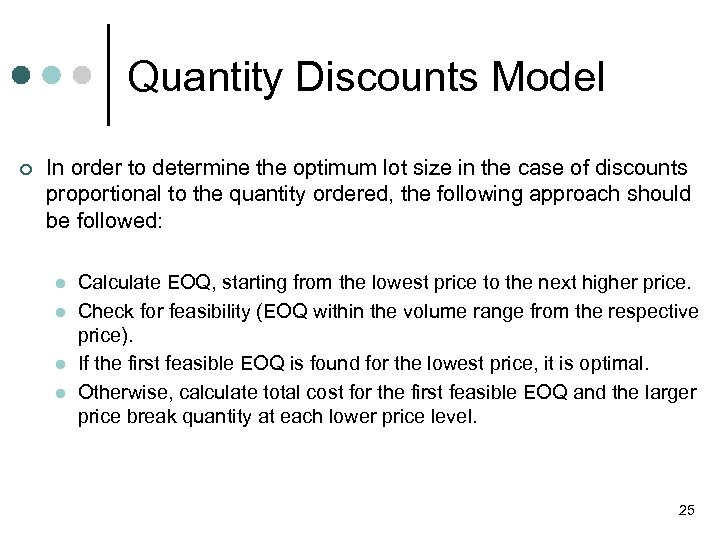Quantity Discounts Model ¢ In order to determine the optimum lot size in the case of discounts proportional to the quantity ordered, the following approach should be followed: l l Calculate EOQ, starting from the lowest price to the next higher price. Check for feasibility (EOQ within the volume range from the respective price). If the first feasible EOQ is found for the lowest price, it is optimal. Otherwise, calculate total cost for the first feasible EOQ and the larger price break quantity at each lower price level. 25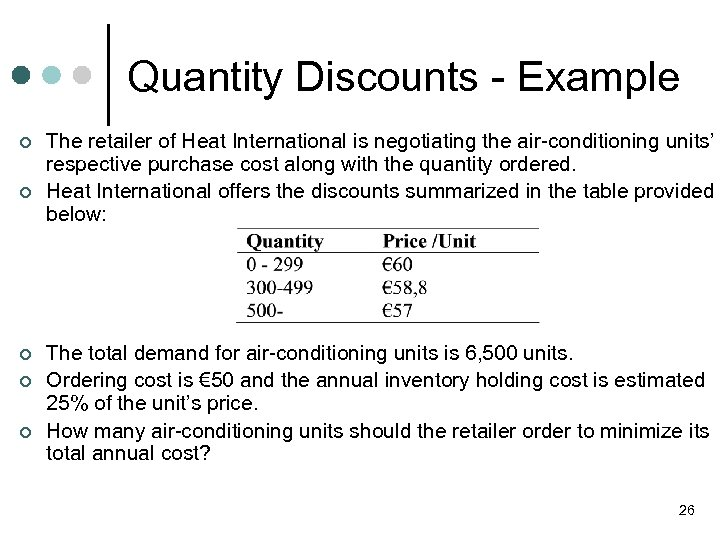Quantity Discounts - Example ¢ ¢ ¢ The retailer of Heat International is negotiating the air-conditioning units’ respective purchase cost along with the quantity ordered. Heat International offers the discounts summarized in the table provided below: The total demand for air-conditioning units is 6, 500 units. Ordering cost is € 50 and the annual inventory holding cost is estimated 25% of the unit’s price. How many air-conditioning units should the retailer order to minimize its total annual cost? 26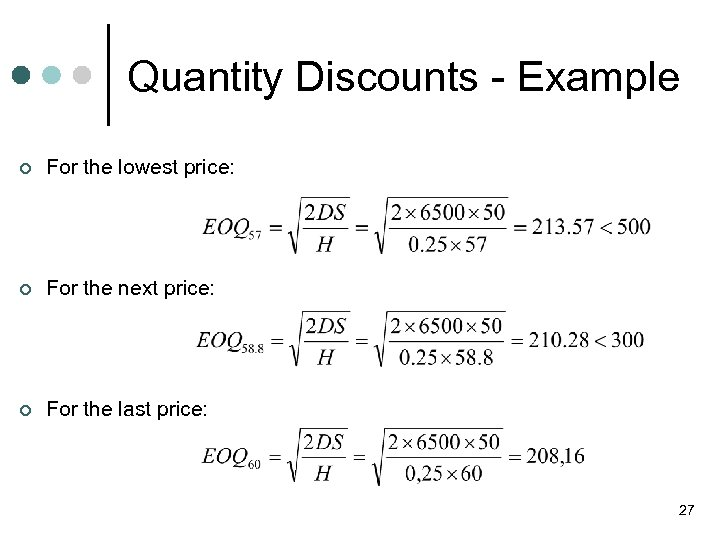Quantity Discounts - Example ¢ For the lowest price: ¢ For the next price: ¢ For the last price: 27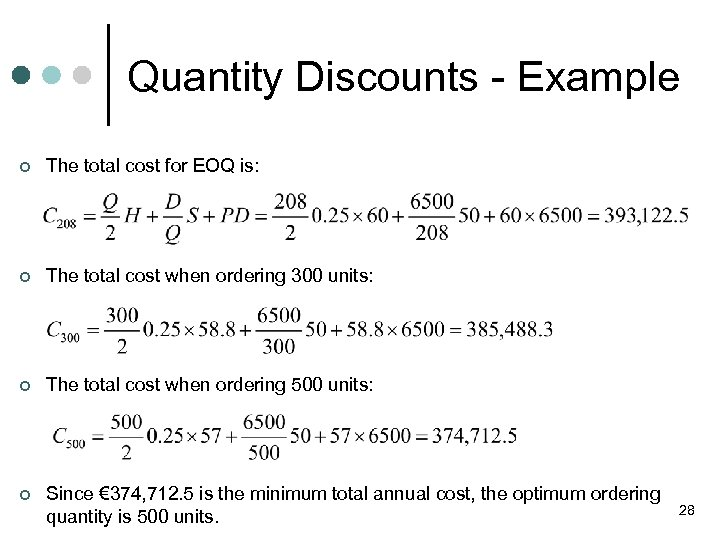Quantity Discounts - Example ¢ The total cost for EOQ is: ¢ The total cost when ordering 300 units: ¢ The total cost when ordering 500 units: ¢ Since € 374, 712. 5 is the minimum total annual cost, the optimum ordering quantity is 500 units. 28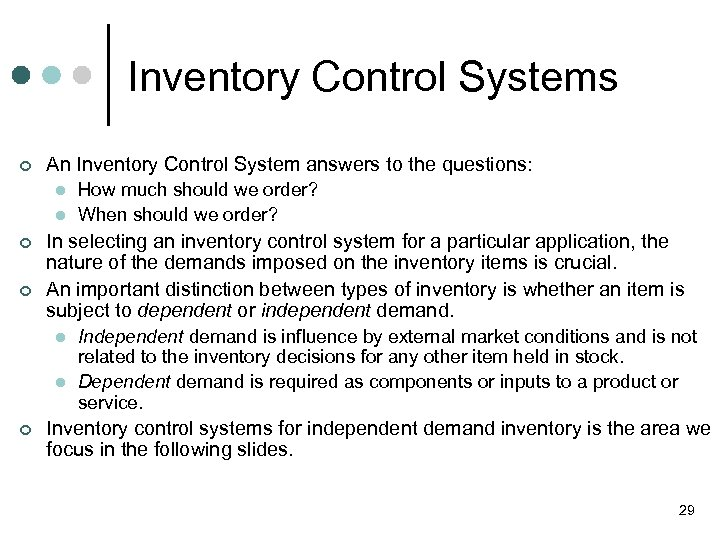Inventory Control Systems ¢ An Inventory Control System answers to the questions: l l ¢ ¢ In selecting an inventory control system for a particular application, the nature of the demands imposed on the inventory items is crucial. An important distinction between types of inventory is whether an item is subject to dependent or independent demand. l l ¢ How much should we order? When should we order? Independent demand is influence by external market conditions and is not related to the inventory decisions for any other item held in stock. Dependent demand is required as components or inputs to a product or service. Inventory control systems for independent demand inventory is the area we focus in the following slides. 29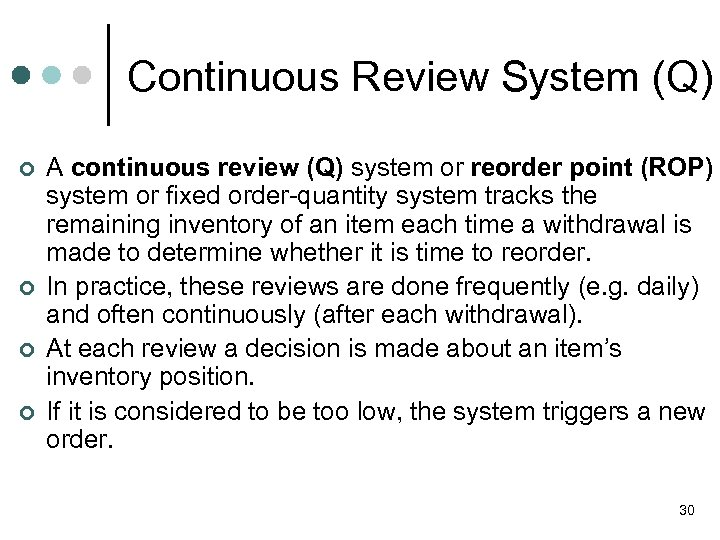Continuous Review System (Q) ¢ ¢ A continuous review (Q) system or reorder point (ROP) system or fixed order-quantity system tracks the remaining inventory of an item each time a withdrawal is made to determine whether it is time to reorder. In practice, these reviews are done frequently (e. g. daily) and often continuously (after each withdrawal). At each review a decision is made about an item’s inventory position. If it is considered to be too low, the system triggers a new order. 30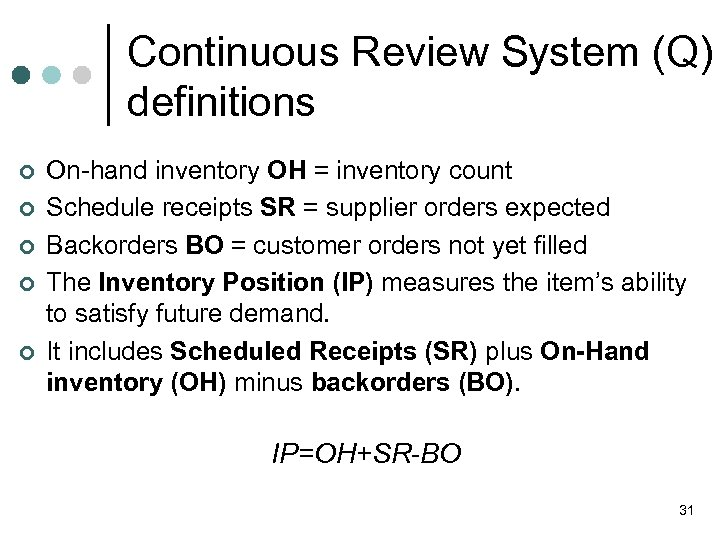Continuous Review System (Q) definitions ¢ ¢ ¢ On-hand inventory OH = inventory count Schedule receipts SR = supplier orders expected Backorders BO = customer orders not yet filled The Inventory Position (IP) measures the item’s ability to satisfy future demand. It includes Scheduled Receipts (SR) plus On-Hand inventory (OH) minus backorders (BO). IP=OH+SR-BO 31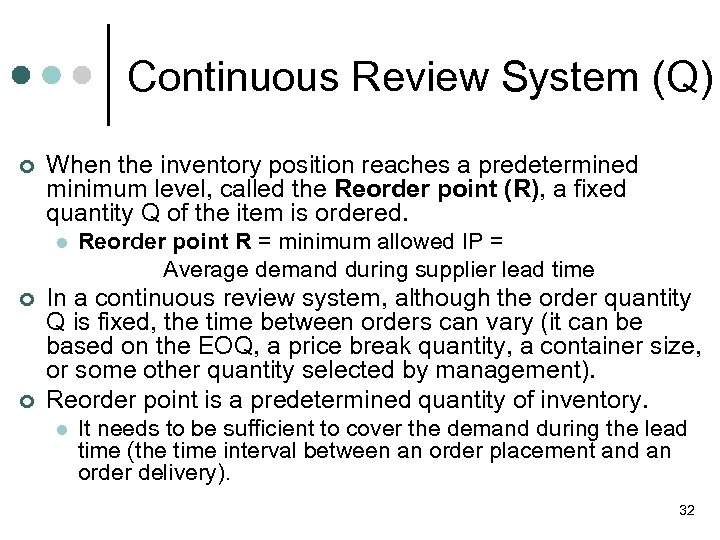Continuous Review System (Q) ¢ When the inventory position reaches a predetermined minimum level, called the Reorder point (R), a fixed quantity Q of the item is ordered. l ¢ ¢ Reorder point R = minimum allowed IP = Average demand during supplier lead time In a continuous review system, although the order quantity Q is fixed, the time between orders can vary (it can be based on the EOQ, a price break quantity, a container size, or some other quantity selected by management). Reorder point is a predetermined quantity of inventory. l It needs to be sufficient to cover the demand during the lead time (the time interval between an order placement and an order delivery). 32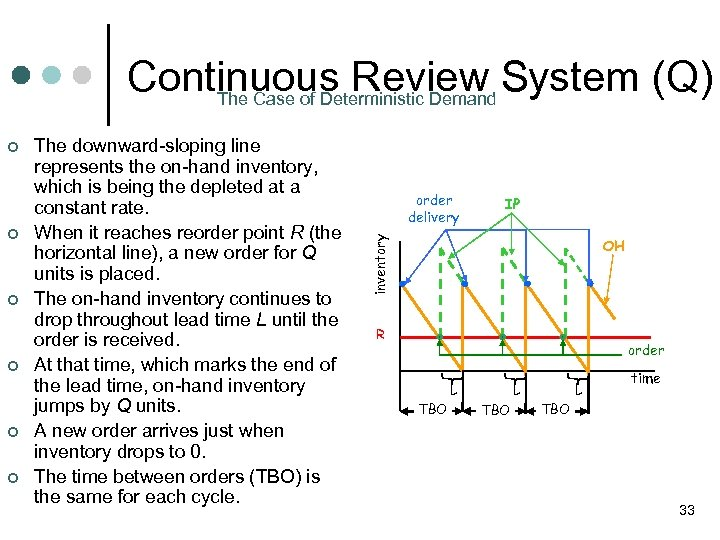Continuous Review System (Q) The Case of Deterministic Demand ¢ ¢ ¢ The downward-sloping line represents the on-hand inventory, which is being the depleted at a constant rate. When it reaches reorder point R (the horizontal line), a new order for Q units is placed. The on-hand inventory continues to drop throughout lead time L until the order is received. At that time, which marks the end of the lead time, on-hand inventory jumps by Q units. A new order arrives just when inventory drops to 0. The time between orders (TBO) is the same for each cycle. order delivery ΙΡ inventory απόθεμα ¢ ΟΗ R order παραγγελία L TBO L time TBO 33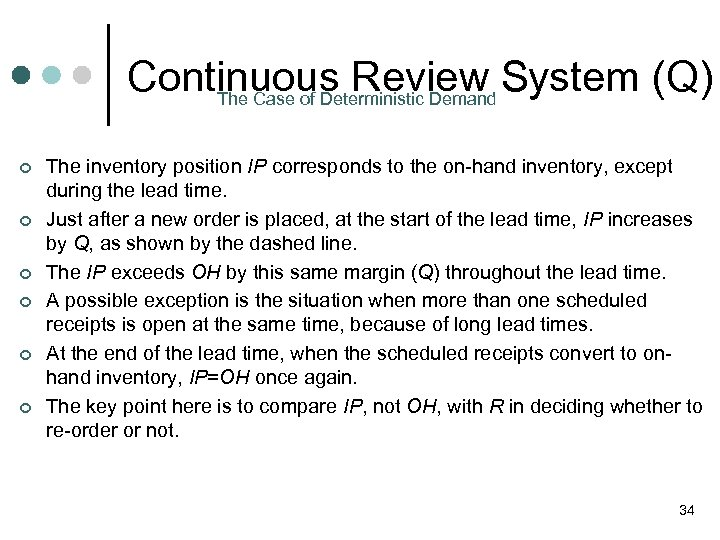Continuous Review System (Q) The Case of Deterministic Demand ¢ ¢ ¢ The inventory position IP corresponds to the on-hand inventory, except during the lead time. Just after a new order is placed, at the start of the lead time, IP increases by Q, as shown by the dashed line. The IP exceeds OH by this same margin (Q) throughout the lead time. A possible exception is the situation when more than one scheduled receipts is open at the same time, because of long lead times. At the end of the lead time, when the scheduled receipts convert to onhand inventory, IP=OH once again. The key point here is to compare IP, not OH, with R in deciding whether to re-order or not. 34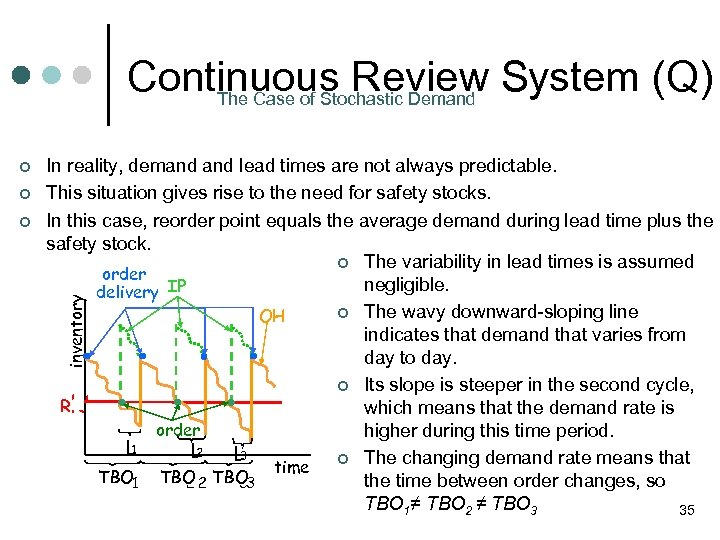Continuous Review System (Q) The Case of Stochastic Demand ¢ ¢ In reality, demand lead times are not always predictable. This situation gives rise to the need for safety stocks. In this case, reorder point equals the average demand during lead time plus the safety stock. ¢ The variability in lead times is assumed order negligible. delivery ΙΡ ¢ The wavy downward-sloping line ΟΗ indicates that demand that varies from day to day. ¢ Its slope is steeper in the second cycle, RR’ ’ which means that the demand rate is order higher during this time period. L 1 2 L 3 L ¢ The changing demand rate means that time ΤΒΟ 1 ΤΒΟ 2 ΤΒΟ 3 the time between order changes, so 2 3 TBO 1≠ TBO 2 ≠ TBO 3 35 inventory ¢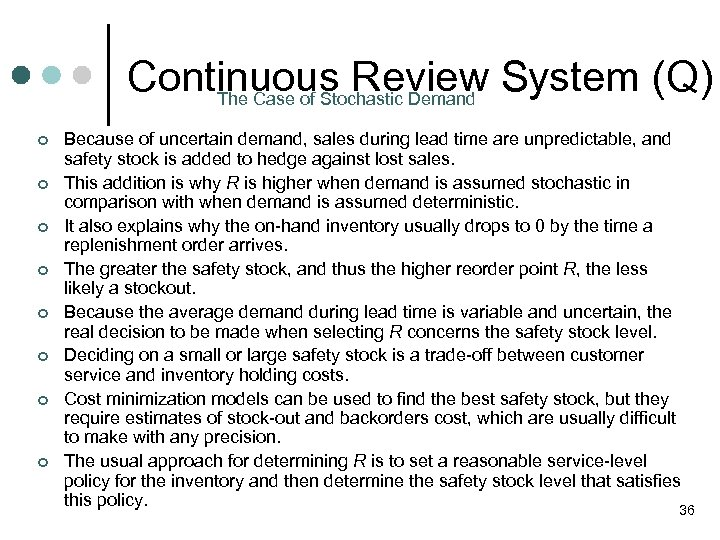Continuous Review System (Q) The Case of Stochastic Demand ¢ ¢ ¢ ¢ Because of uncertain demand, sales during lead time are unpredictable, and safety stock is added to hedge against lost sales. This addition is why R is higher when demand is assumed stochastic in comparison with when demand is assumed deterministic. It also explains why the on-hand inventory usually drops to 0 by the time a replenishment order arrives. The greater the safety stock, and thus the higher reorder point R, the less likely a stockout. Because the average demand during lead time is variable and uncertain, the real decision to be made when selecting R concerns the safety stock level. Deciding on a small or large safety stock is a trade-off between customer service and inventory holding costs. Cost minimization models can be used to find the best safety stock, but they require estimates of stock-out and backorders cost, which are usually difficult to make with any precision. The usual approach for determining R is to set a reasonable service-level policy for the inventory and then determine the safety stock level that satisfies this policy. 36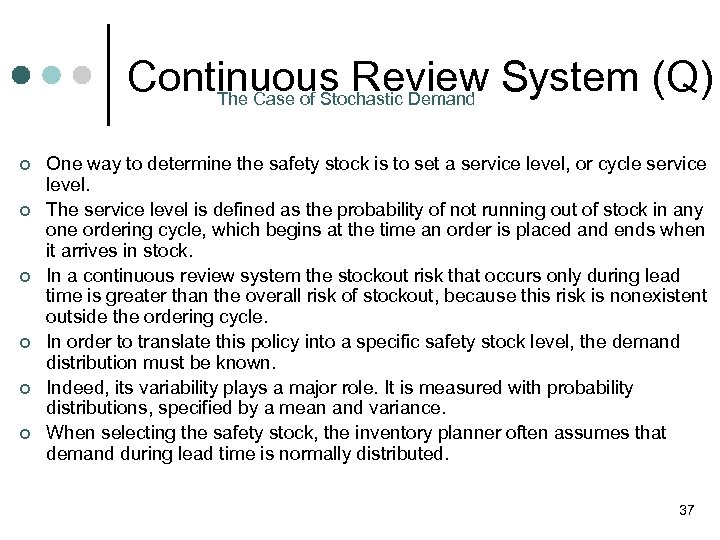Continuous Review System (Q) The Case of Stochastic Demand ¢ ¢ ¢ One way to determine the safety stock is to set a service level, or cycle service level. The service level is defined as the probability of not running out of stock in any one ordering cycle, which begins at the time an order is placed and ends when it arrives in stock. In a continuous review system the stockout risk that occurs only during lead time is greater than the overall risk of stockout, because this risk is nonexistent outside the ordering cycle. In order to translate this policy into a specific safety stock level, the demand distribution must be known. Indeed, its variability plays a major role. It is measured with probability distributions, specified by a mean and variance. When selecting the safety stock, the inventory planner often assumes that demand during lead time is normally distributed. 37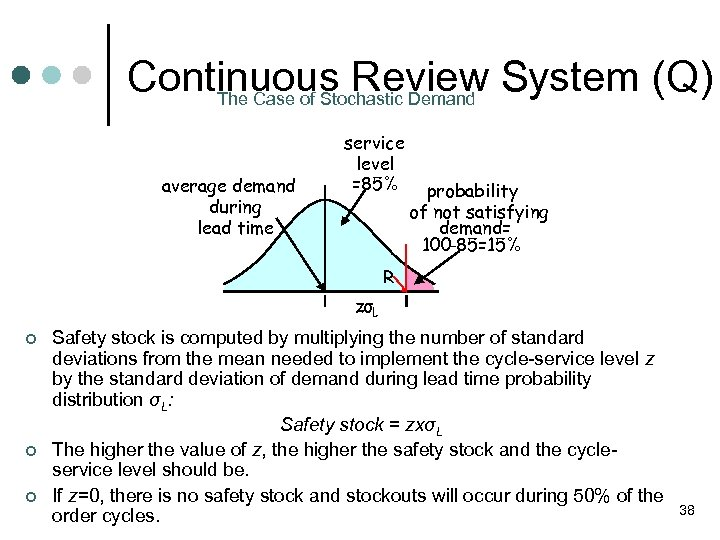Continuous Review System (Q) The Case of Stochastic Demand average demand during lead time service level =85% probability of not satisfying demand= 100 -85=15% R zσL ¢ ¢ ¢ Safety stock is computed by multiplying the number of standard deviations from the mean needed to implement the cycle-service level z by the standard deviation of demand during lead time probability distribution σL: Safety stock = zxσL The higher the value of z, the higher the safety stock and the cycleservice level should be. If z=0, there is no safety stock and stockouts will occur during 50% of the order cycles. 38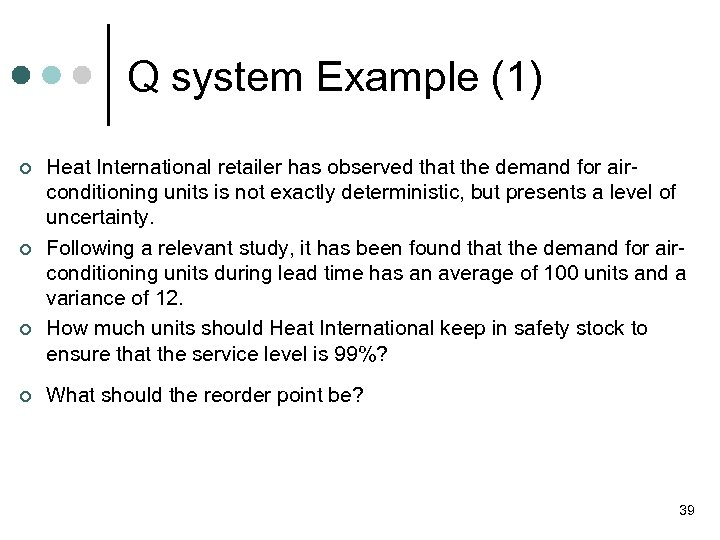Q system Example (1) ¢ ¢ Heat International retailer has observed that the demand for airconditioning units is not exactly deterministic, but presents a level of uncertainty. Following a relevant study, it has been found that the demand for airconditioning units during lead time has an average of 100 units and a variance of 12. How much units should Heat International keep in safety stock to ensure that the service level is 99%? What should the reorder point be? 39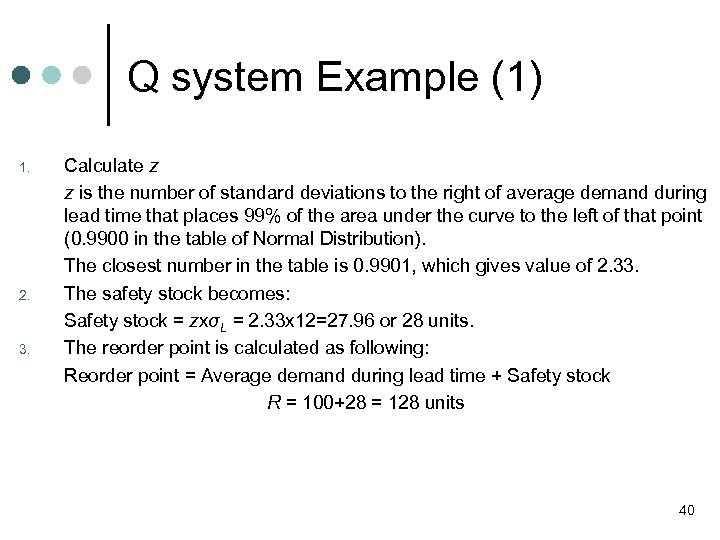Q system Example (1) 1. 2. 3. Calculate z z is the number of standard deviations to the right of average demand during lead time that places 99% of the area under the curve to the left of that point (0. 9900 in the table of Normal Distribution). The closest number in the table is 0. 9901, which gives value of 2. 33. The safety stock becomes: Safety stock = zxσL = 2. 33 x 12=27. 96 or 28 units. The reorder point is calculated as following: Reorder point = Average demand during lead time + Safety stock R = 100+28 = 128 units 40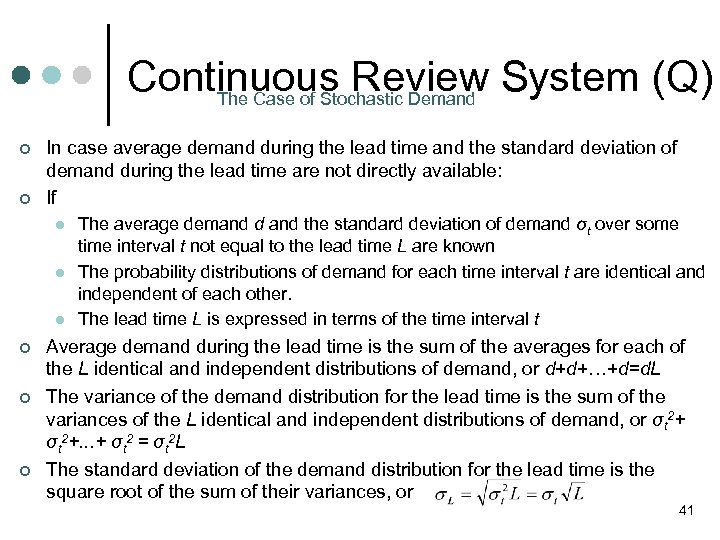Continuous Review System (Q) The Case of Stochastic Demand ¢ ¢ In case average demand during the lead time and the standard deviation of demand during the lead time are not directly available: If l l l ¢ ¢ ¢ The average demand d and the standard deviation of demand σt over some time interval t not equal to the lead time L are known The probability distributions of demand for each time interval t are identical and independent of each other. The lead time L is expressed in terms of the time interval t Average demand during the lead time is the sum of the averages for each of the L identical and independent distributions of demand, or d+d+…+d=d. L The variance of the demand distribution for the lead time is the sum of the variances of the L identical and independent distributions of demand, or σt 2+. . . + σt 2 = σt 2 L The standard deviation of the demand distribution for the lead time is the square root of the sum of their variances, or 41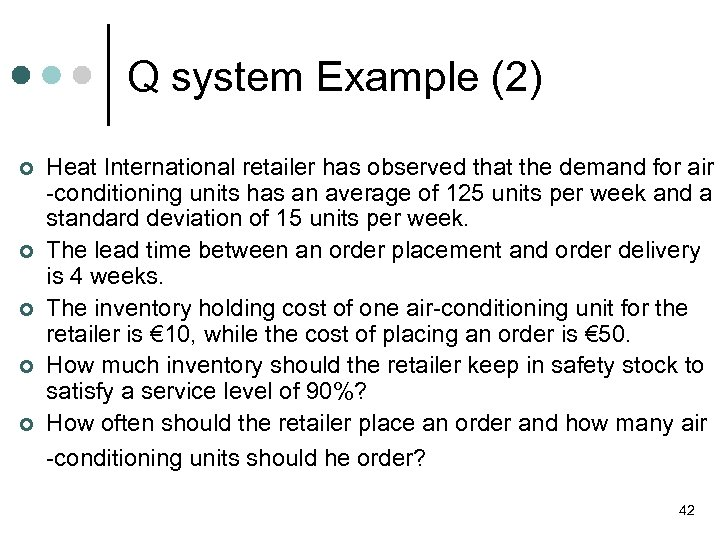Q system Example (2) ¢ ¢ ¢ Heat International retailer has observed that the demand for air -conditioning units has an average of 125 units per week and a standard deviation of 15 units per week. The lead time between an order placement and order delivery is 4 weeks. The inventory holding cost of one air-conditioning unit for the retailer is € 10, while the cost of placing an order is € 50. How much inventory should the retailer keep in safety stock to satisfy a service level of 90%? How often should the retailer place an order and how many air -conditioning units should he order? 42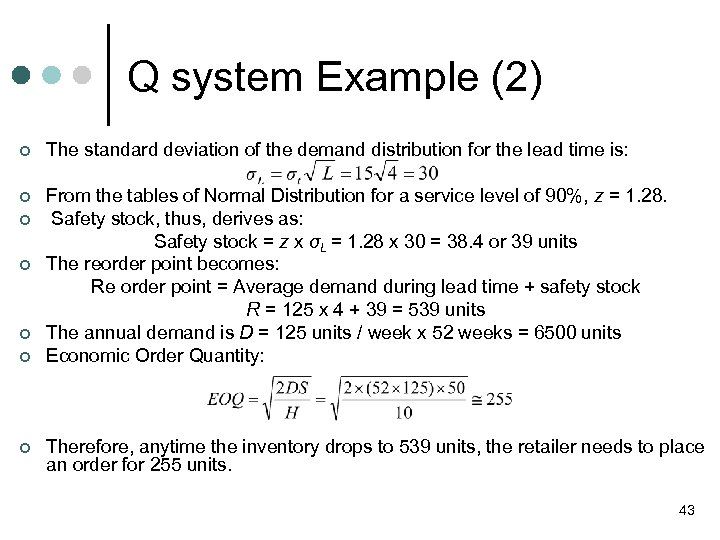Q system Example (2) ¢ The standard deviation of the demand distribution for the lead time is: ¢ From the tables of Normal Distribution for a service level of 90%, z = 1. 28. Safety stock, thus, derives as: Safety stock = z x σL = 1. 28 x 30 = 38. 4 or 39 units The reorder point becomes: Re order point = Average demand during lead time + safety stock R = 125 x 4 + 39 = 539 units The annual demand is D = 125 units / week x 52 weeks = 6500 units Economic Order Quantity: ¢ ¢ ¢ Therefore, anytime the inventory drops to 539 units, the retailer needs to place an order for 255 units. 43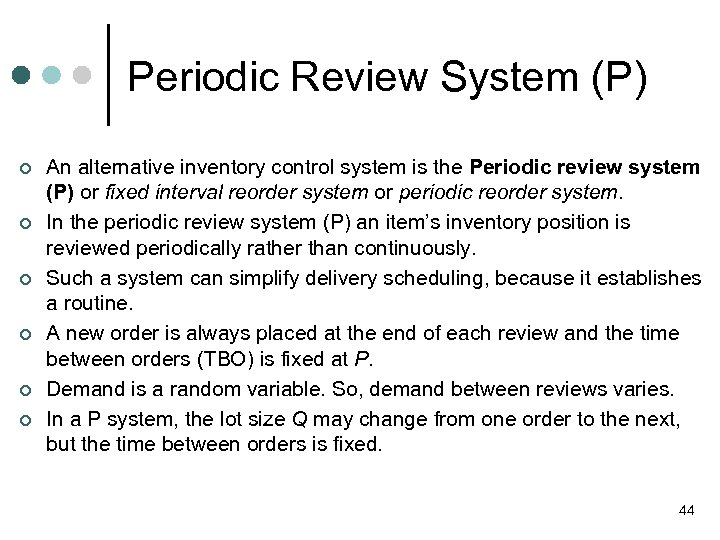Periodic Review System (P) ¢ ¢ ¢ An alternative inventory control system is the Periodic review system (P) or fixed interval reorder system or periodic reorder system. In the periodic review system (P) an item’s inventory position is reviewed periodically rather than continuously. Such a system can simplify delivery scheduling, because it establishes a routine. A new order is always placed at the end of each review and the time between orders (TBO) is fixed at P. Demand is a random variable. So, demand between reviews varies. In a P system, the lot size Q may change from one order to the next, but the time between orders is fixed. 44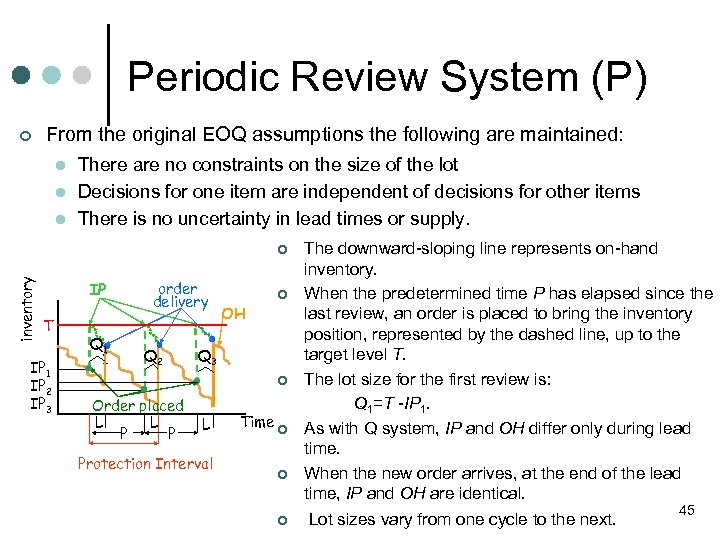Periodic Review System (P) ¢ From the original EOQ assumptions the following are maintained: l l l There are no constraints on the size of the lot Decisions for one item are independent of decisions for other items There is no uncertainty in lead times or supply. inventory ¢ ΙΡ Τ ΙΡ 1 ΙΡ 2 ΙΡ 3 Q 1 1 order delivery Q 2 ¢ ΟΗ Q 3 ¢ Order placed L L P P L Protection Interval Time ¢ ¢ ¢ The downward-sloping line represents on-hand inventory. When the predetermined time P has elapsed since the last review, an order is placed to bring the inventory position, represented by the dashed line, up to the target level T. The lot size for the first review is: Q 1=T -IP 1. As with Q system, IP and OH differ only during lead time. When the new order arrives, at the end of the lead time, IP and OH are identical. Lot sizes vary from one cycle to the next. 45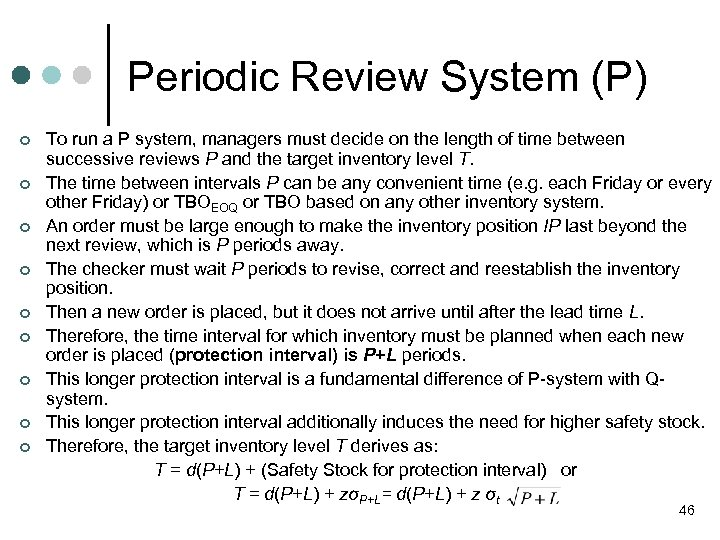Periodic Review System (P) ¢ ¢ ¢ ¢ ¢ To run a P system, managers must decide on the length of time between successive reviews P and the target inventory level T. The time between intervals P can be any convenient time (e. g. each Friday or every other Friday) or TBOEOQ or TBO based on any other inventory system. An order must be large enough to make the inventory position IP last beyond the next review, which is P periods away. The checker must wait P periods to revise, correct and reestablish the inventory position. Then a new order is placed, but it does not arrive until after the lead time L. Therefore, the time interval for which inventory must be planned when each new order is placed (protection interval) is P+L periods. This longer protection interval is a fundamental difference of P-system with Qsystem. This longer protection interval additionally induces the need for higher safety stock. Therefore, the target inventory level T derives as: T = d(P+L) + (Safety Stock for protection interval) or T = d(P+L) + zσP+L= d(P+L) + z σt 46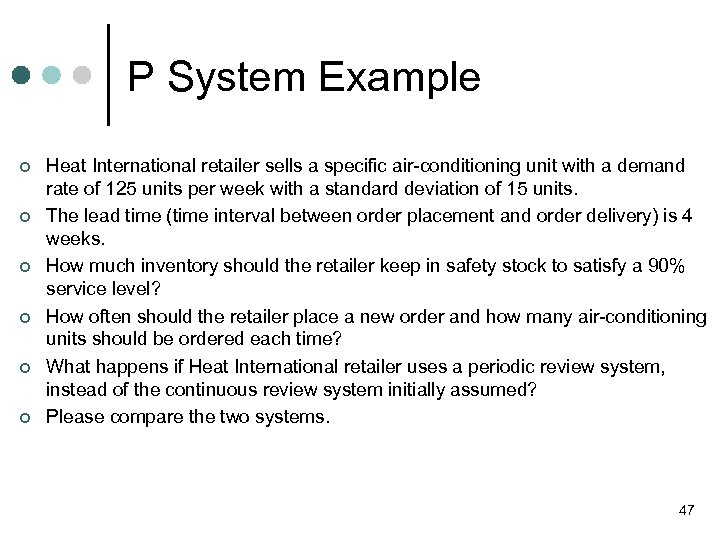P System Example ¢ ¢ ¢ Heat International retailer sells a specific air-conditioning unit with a demand rate of 125 units per week with a standard deviation of 15 units. The lead time (time interval between order placement and order delivery) is 4 weeks. How much inventory should the retailer keep in safety stock to satisfy a 90% service level? How often should the retailer place a new order and how many air-conditioning units should be ordered each time? What happens if Heat International retailer uses a periodic review system, instead of the continuous review system initially assumed? Please compare the two systems. 47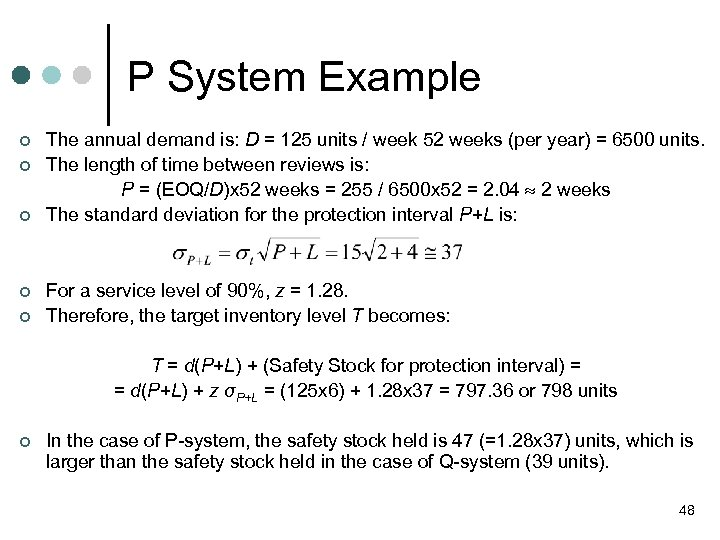P System Example ¢ ¢ ¢ The annual demand is: D = 125 units / week 52 weeks (per year) = 6500 units. The length of time between reviews is: P = (EOQ/D)x 52 weeks = 255 / 6500 x 52 = 2. 04 2 weeks The standard deviation for the protection interval P+L is: For a service level of 90%, z = 1. 28. Therefore, the target inventory level T becomes: T = d(P+L) + (Safety Stock for protection interval) = = d(P+L) + z σP+L = (125 x 6) + 1. 28 x 37 = 797. 36 or 798 units ¢ In the case of P-system, the safety stock held is 47 (=1. 28 x 37) units, which is larger than the safety stock held in the case of Q-system (39 units). 48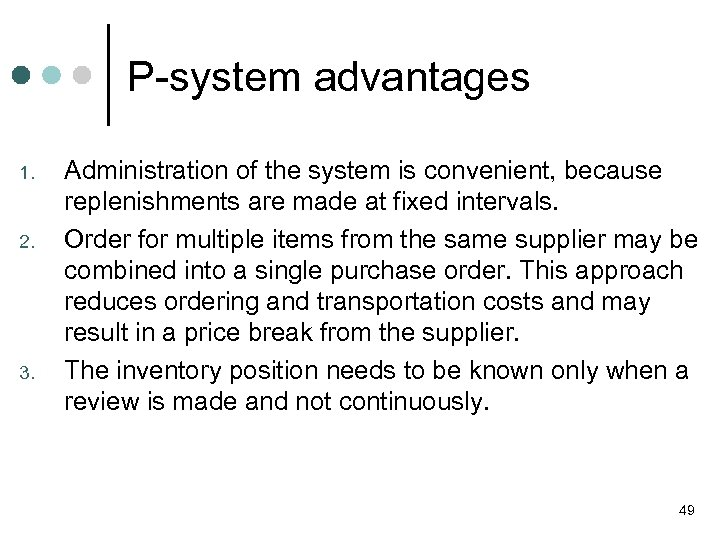P-system advantages 1. 2. 3. Administration of the system is convenient, because replenishments are made at fixed intervals. Order for multiple items from the same supplier may be combined into a single purchase order. This approach reduces ordering and transportation costs and may result in a price break from the supplier. The inventory position needs to be known only when a review is made and not continuously. 49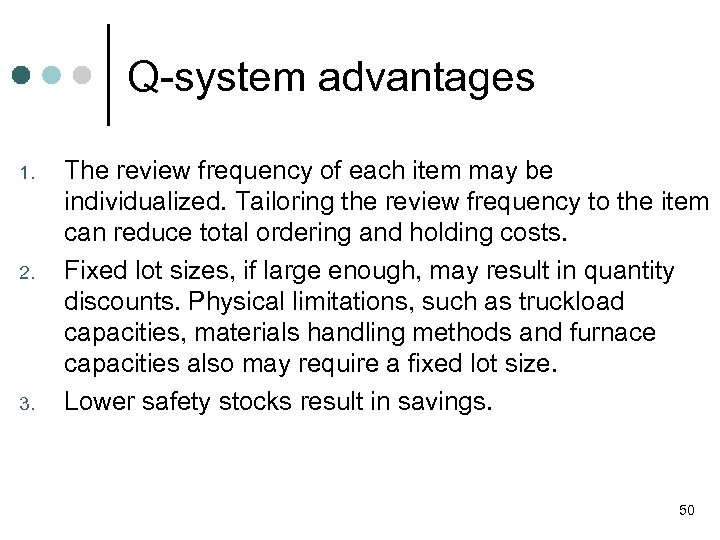Q-system advantages 1. 2. 3. The review frequency of each item may be individualized. Tailoring the review frequency to the item can reduce total ordering and holding costs. Fixed lot sizes, if large enough, may result in quantity discounts. Physical limitations, such as truckload capacities, materials handling methods and furnace capacities also may require a fixed lot size. Lower safety stocks result in savings. 50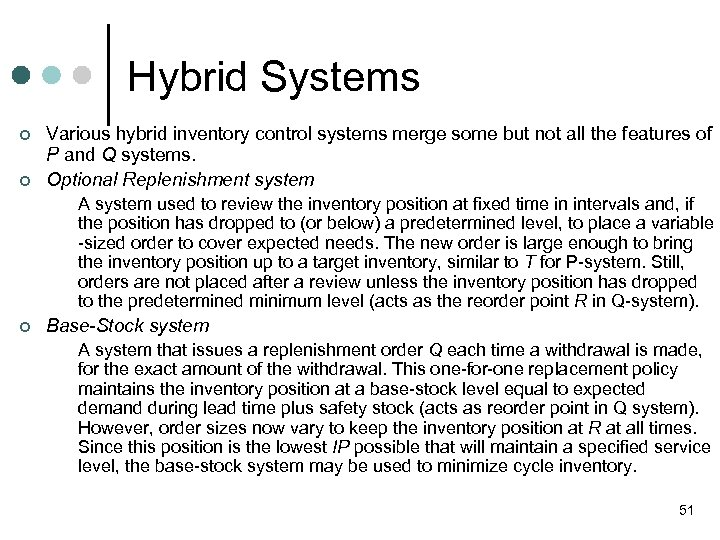Hybrid Systems ¢ ¢ Various hybrid inventory control systems merge some but not all the features of P and Q systems. Optional Replenishment system A system used to review the inventory position at fixed time in intervals and, if the position has dropped to (or below) a predetermined level, to place a variable -sized order to cover expected needs. The new order is large enough to bring the inventory position up to a target inventory, similar to T for P-system. Still, orders are not placed after a review unless the inventory position has dropped to the predetermined minimum level (acts as the reorder point R in Q-system). ¢ Base-Stock system A system that issues a replenishment order Q each time a withdrawal is made, for the exact amount of the withdrawal. This one-for-one replacement policy maintains the inventory position at a base-stock level equal to expected demand during lead time plus safety stock (acts as reorder point in Q system). However, order sizes now vary to keep the inventory position at R at all times. Since this position is the lowest IP possible that will maintain a specified service level, the base-stock system may be used to minimize cycle inventory. 51